# Fraction Spinners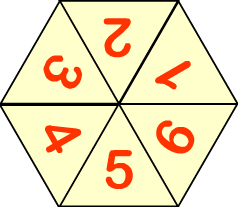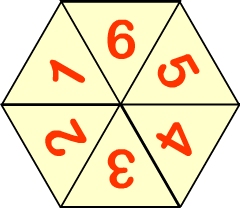What do you know about the fraction ?

Stand up if it is a whole number.

Put a hand up if it can be simplified.

Hum if it is an improper fraction.

Sway from side to side if it is greater than a half.

#### Shine + Write | Transum Mathematics

This is a visual aid designed to be projected onto a whiteboard for whole class exposition. Click on the blue buttons to hide statements which would not be appropriate for your class.

### More Fraction Activities and Resources

#### Fraction Wall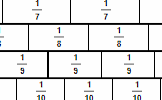The traditional fraction wall diagram showing the relationship between simple fractions. Click the bricks to change their colour.

www.transum.org/go/?to=wall

#### Equivalent Fractions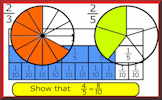Practise finding equivalent fractions numerically and in fraction diagrams. A colourful was to learn about the properties of fractions.

www.transum.org/go/?to=equivalent

#### Fraction Line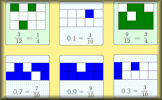Arrange the fractions in order from smallest to largest. Three different drag-and-drop challenges requiring an understanding of fractions.

www.transum.org/go/?to=fractions

#### Vinculum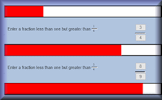Find fractions which are larger than the previous fraction but less than one. A worthwhile investigation of strategies.

www.transum.org/go/?to=vinculum

#### Fractions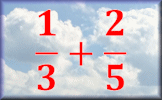Do these four operations fractions calculations using pen and paper to help with the working out.

www.transum.org/go/?to=fract

#### Fractions Decimals Percentages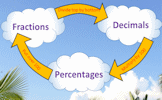Revise the methods for converting fractions to decimals and percentages.

www.transum.org/go/?to=fracdecpercent

Fractions Index

"It's a good feature to let you take out particular instructions either for weaker students or to gradually increase the difficulty of the task. Spot on!"

EHC, The Market Weighton School
Monday, November 15, 2010

Do you have any comments? It is always useful to receive feedback and helps make this free resource even more useful for those learning Mathematics anywhere in the world. Click here to enter your comments.For All: